NEET  >  Previous Year Questions (2014-22): Physical World, Units & Measurement

# Previous Year Questions (2014-22): Physical World, Units & Measurement - Notes | Study Physics Class 11 - NEET

 1 Crore+ students have signed up on EduRev. Have you?

Q.1. The dimensions [MLT–2A–2 ] belong to the              
(1) Self-inductance
(2) Magnetic permeability
(3) Electric permittivity
(4) Magnetic flux

The dimensional formula of magnetic permeability is [MLT–2A–2 ]

Q.2. The area of a rectangular field (in m2 ) of length 55.3 m and breadth 25 m after rounding off the value for correct significant digits is                           
(1) 1382

(2) 1382.5
(3) 14 * 10
(4) 138 * 10
1

= 55.3 × 25 m2
= 1382.5 m2
= 14 × 102 m2
(Rounding off of two significant figures)

Q.3. Match List-I with List-II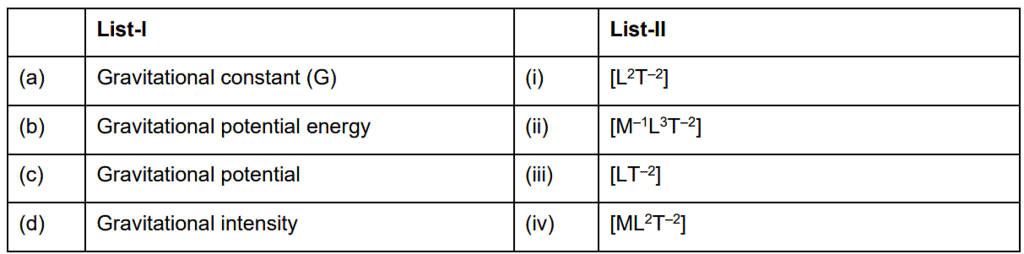Choose the correct answer from the options given below                 
(1) (a) - (ii), (b) - (iv), (c) - (i), (d) - (iii)

(2) (a) - (ii), (b) - (iv), (c) - (iii), (d) - (i)

(3) (a) - (iv), (b) - (ii), (c) - (i), (d) - (iii)

(4) (a) - (ii), (b) - (i), (c) - (iv), (d) - (iii)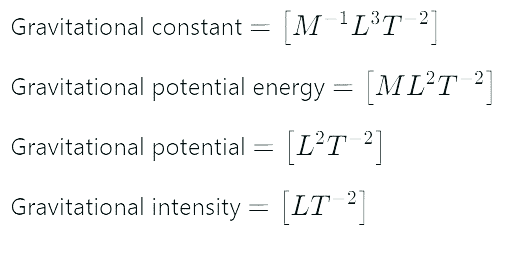Q.4. Plane angle and solid angle have
(1) Dimensions but no units
(2) No units and no dimensions
(3) Both units and dimensions
(4) Units but no dimensions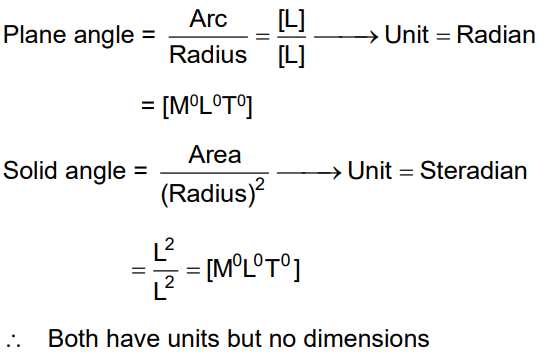Q.5. A Screw Guage gives the following readings when used to measure the diameter of a wire.
Main scale reading = 0.0 mm
Circular scale reading = 52 divisions
Given that: 1 mm on the main scale corresponds to 100 divisions of the circular scale.
The diameter of the wire from the above data is:

A: 0.026 cm
B: 0.005 cm
C: 0.52 cm
D: 0.052 cm
Ans:
D
Solution: Diameter of wire,Q.6. If force [F], acceleration [A] and time [T] are chosen as the fundamental physical quantities. Find the dimensions of energy.    
A: [F][A][T-1]
B: [F][A-1][T]
C: [F][A][T]
D: [F][A][T2]
Ans:
D
Solution:Q.7. If E and G respectively denote energy and gravitational constant, then E/G has the dimensions of :    
A: [M] [L0] [T0]
B: [M2] [L–2] [T–1]
C: [M2] [L–1] [T0]
D: [M] [L–1] [T–1]
Ans:
C
Solution:

E = energy = [ML2T–2]
G = Gravitational constant = [M–1L3T–2]
So,
E/G = [E]/[G]
= ML2T–2/M–1L3T–2
= [M2L-1T0]

Q.8. Taking into account of the significant figures, what is the value of 9.99 m-0.0099 m?    
A: 9.980 m
B: 9.9 m
C: 9.9801 m
D: 9.98 m

Ans: D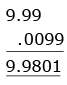As per rule
least no. of place in decimal portion of any number 9.98

Q.9. In an experiment, the percentage of error occurred in the measurement of physical quantities A, B, C and D are 1%, 2%, 3% and 4% respectively. Then the maximum percentage of error in the measurement X, where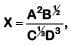will be     
A: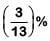B: 16 %
C: -10 %
D: 10 %
Ans:
B
Given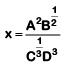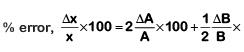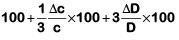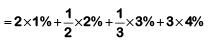= 2% + 1%+ 1%+ 12%= 16%

Q.10. A student measured the diameter of a small steel ball using a screw gauge of least count 0.001 cm. The main scale reading is 5 mm and zero of circular scale division coincides with 25 divisions above the reference level. If screw gauge has a zero error of – 0.004 cm, the correct diameter of the ball is :-    
A: 0.521 cm
B: 0.525 cm
C: 0.053 cm
D: 0.529 cm

Ans: D
Solution:
= MSR + VSR × LC + zero error
= 0.5 cm + 25 × 0.001 cm + 0.004 cm
= 0.529 cm

Q.11. A physical quantity of the dimensions of length that can be formed out of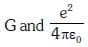is velocity of light, G is universal constant of gravitation and e is charge] :-    

A: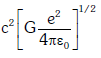B: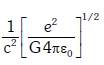C: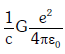D: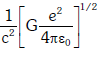Ans: D
Solution: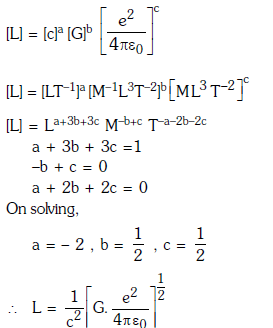Q.12. If energy (E), velocity (V) and time (T) are chosen as the fundamental quantities, the dimensional formula of surface tension will be:    
A: [E-2 V-1 T-3]
B: [E V-2 T-1]
C: [E V-1 T-2]
D: [E V-2 T-2]
Ans:
D
Solution: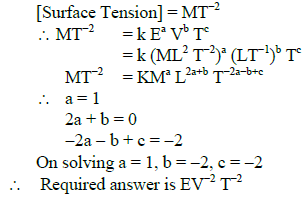Q.13. If force (F), velocity (V) and time(T) are taken as fundamental units, then the dimensions of mass are:    
A: [F V−1 T−1]
B: [F V−1 T]
C: [F V T−1]
D: [F V T−2]
Ans:
B
Solution: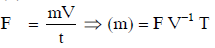The document Previous Year Questions (2014-22): Physical World, Units & Measurement - Notes | Study Physics Class 11 - NEET is a part of the NEET Course Physics Class 11.
All you need of NEET at this link: NEET

## Physics Class 11

127 videos|464 docs|210 tests
 Use Code STAYHOME200 and get INR 200 additional OFF

## Physics Class 11

127 videos|464 docs|210 tests

### How to Prepare for NEET

Read our guide to prepare for NEET which is created by Toppers & the best Teachers

Track your progress, build streaks, highlight & save important lessons and more!

,

,

,

,

,

,

,

,

,

,

,

,

,

,

,

,

,

,

,

,

,

,

,

,

;